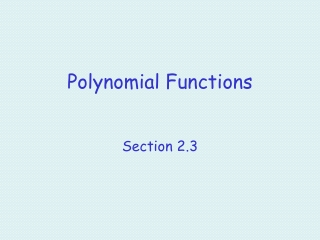DownloadDownload PresentationPolynomial Functions

# Polynomial Functions

Télécharger la présentation## Polynomial Functions

- - - - - - - - - - - - - - - - - - - - - - - - - - - E N D - - - - - - - - - - - - - - - - - - - - - - - - - - -
##### Presentation Transcript

1. Polynomial Functions Section 2.3

2. Objectives • Find the x-intercepts and y-intercept of a polynomial function. • Describe the end behaviors of a polynomial function. • Write the equation of a polynomial function given the zeros and a point on the function. • Determine the minimal degree of a polynomial given its graph. • Solve a word problem involving polynomial function.

3. Objectives • Use a graphing utility to find a local maximum or local minimum of a polynomial function. • Use a graphing utility to find the absolute maximum or absolute minumum of a polynomial function. • Use a graphing utility to find the intersection points of the graphs of two polynomials.

4. Vocabulary • polynomial function • degree • leading coefficient • end behavior • repeated zero • multiplicity • local minimum • local maximum • absolute minimum • absolute maximum

5. Graph each of the following: positive leading coefficient and even degree

6. Graph each of the following: negative leading coefficient and even degree

7. Graph each of the following: positive leading coefficient and odd degree

8. Graph each of the following: negative leading coefficient and odd degree

9. For the function • Find the x-intercept(s). • Find the y-intercept(s). • Describe the end behaviors.

10. For the function • Find the x-intercept(s). • Find the y-intercept(s). • Describe the end behaviors.

11. Find a possible formula for the polynomial of degree 4 that has a root of multiplicity 2 at x = 2 and roots of multiplicity 1 at x = 0 and x = -2 that goes through the point (5, 63).

12. What is the smallest possible degree of the polynomial whose graph is given below.

13. A box without a lid is constructed from a 36 inch by 36 inch piece of cardboard by cutting x inch squares from each corner and folding up the sides. • Determine the volume of the box as a function of the variable x. • Use a graphing utility to approximate the values of x that produce a volume of 3280.5 cubic inches.

14. Consider the function: Find the absolute maximum and absolute minimum of the graph.